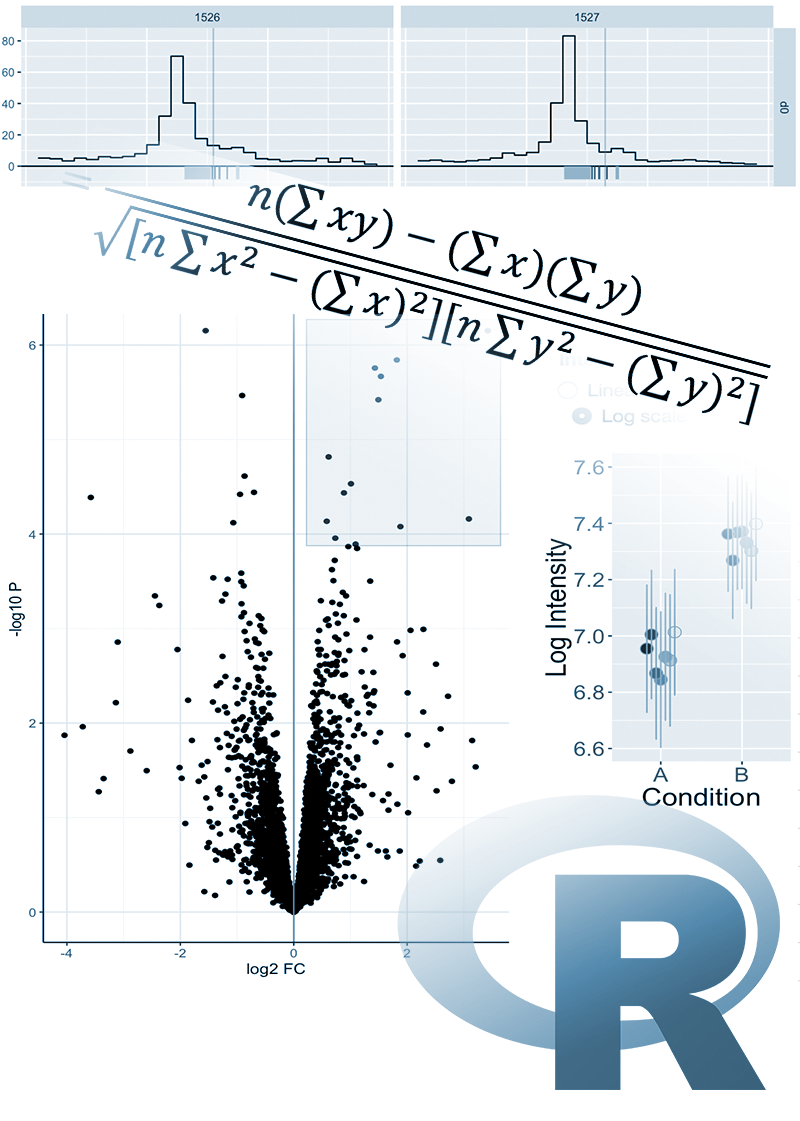## Module Overview

Statistical tests are used to determine whether the observed effect is real or merely occurred by chance. An outcome of a statistical test is a p-value, the probability of getting the observed, or stronger, result by chance. P-values are essential for data analysis, but alas, they are frequently misunderstood and misused, often considered as the ultimate answer, while they only form a small part of the bigger puzzle. This ultimately results in the publication of erroneous and irreproducible results.

Every numerical value in experimental biology, measured or derived, has an inherent uncertainty, or error. Understanding, interpretation and estimating errors can be hard.

This series of lectures explores a range of statistical tests, when their use is appropriate and correctly interpreting their results. Additionally, it will explain the meaning of errors and show how these can be calculated in typical biological applications. The topics covered by the lectures are as follows:

1. Probability distributions: Discrete and continuous random variables; probability distribution; normal, Poisson and binomial distribution
2. Errors and statistical estimators: Measurement and random errors; population and sample; statistical estimators; standard deviation and standard error
3. Confidence intervals 1: What is confidence; sampling distribution; confidence interval of the mean and median
4. Confidence intervals 2: Confidence interval for count data, correlation and proportion; bootstrapping
5. Data presentation: How to make a good plot; lines and symbols; logarithmic plots; error bars; box plots; bar plots; more about bar plots; you will be sick of bar plots; quoting numbers and errors
6. Statistical hypothesis testing: Null hypothesis, statistical test and p-value; Fisher’s test;
7. Contigency tables: Chi-square test, G-test; goodness-of-fit and independence tests;
8. t-test: One- and two-sample t-test, paired t-test; variance comparison
9. ANOVA: One- and two-way ANOVA; F-test, Levene’s test for equality of variances, Tukey’s post-hoc test; time-course experiments
10. Non-parametric tests: Mann-Whitney, Wilcoxon signed-rank and Kruskal-Wallis tests; Kolmogorov-Smirnov [extra: permutation and bootstrap tests, Monte-Carlo chi-square test]
11. Statistical power: Effect size, power in t-test, power in ANOVA
12. Multiple test corrections: Family-wise error rate, false discovery rate, Holm-Bonferroni limit, Benjamini-Hochberg limit, Storey method
13. Linear models: Simple linear regression, qualitative predictors, design matrix, fit coefficients, p-values. Multiple variables.
14. What’s wrong with p-values?: Spoiler: a lot!

Pre-registration is not required to attend this lecture series.

## Learning Outcomes

After completing this module, participants will:
• Understand the meaning of error in a mathematical context
• Understand how to determine the error associated with a dataset
• Understand the use of confidence intervals to represent the range in which an error may lie
• Understand how to represent error when reporting results
• Be able to select an appropriate statistical test to apply
• Understand how to interpret p-values and false discovery rates
• Understand when use of a p-value is appropriate

## Prerequisite Modules/Knowledge

Attendance of OPD workshop ‘An Introduction to the Elements of Modern Statistical Modelling Using the Language R’ workshop is highly recommended.

## Course Materials

Videos and slides of the 2020 lecture series are available below.
Lecture Slides Video
Probability distributions View slides Watch video
Measurement errors; statistical estimators View slides Watch video
Confidence intervals 1 View slides Watch video
Confidence intervals 2 View slides Watch video
Data presentation View slides Watch video
Statistical hypothesis testing View slides Watch video
Contingency tables View slides Watch video
t-test View slides Watch video
ANOVA View slides Watch video
Non-parametric tests View slides Watch video
Statistical power View slides Watch video
Multiple test corrections View slides Watch video
Linear Models View slides Watch video
What’s wrong with p-values? View slides Watch video